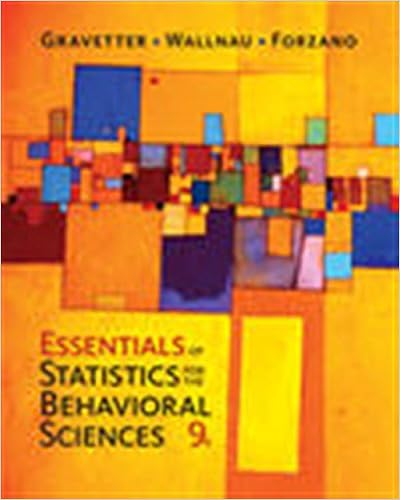# Q10 based on your evaluation of the null in q8 and

• Homework Help
• 6
• 61% (23) 14 out of 23 people found this document helpful

This preview shows page 3 - 5 out of 6 pages.

##### We have textbook solutions for you!
The document you are viewing contains questions related to this textbook.The document you are viewing contains questions related to this textbook.
Chapter 4 / Exercise 2
Essentials of Statistics for the Behavioral Sciences
Gravetter/WallnauExpert Verified
Q10: Based on your evaluation of the null in Q8 and your conclusion is Q9, as a researcher you would be more concerned with a A. Type I statistical error B. Type II statistical error Calculate the 99% confidence interval. Steps: Q11: The mean you will use for this calculation is A. 5.5 B. 6.3
Q12: What is the new critical value you will use for this calculation? Q13: As you know, two values will be required to complete the following equation: __________ __________ The following 4 questions (Q14 to Q17) are based on the following situation: If α = .10, and β = .30, complete the following questions by inserting the appropriate probability of each. Q14: The statistical decision is to reject the null, and H 0 is really true (ie: a Type I error) Q15: The statistical decision is to fail to reject null, and H 0 is really true (ie: a correct decision) Q16: The statistical decision is to reject the null, and H 0 is really false (ie: Power)
##### We have textbook solutions for you!
The document you are viewing contains questions related to this textbook.The document you are viewing contains questions related to this textbook.
Chapter 4 / Exercise 2
Essentials of Statistics for the Behavioral Sciences
Gravetter/WallnauExpert Verified
Q17: The statistical decision is to fail to reject the null, and H 0 is really false (ie a Type II error) The following 14 questions (Q18 to Q31) are based on the following example: A researcher wants to determine whether high school students who attend an SAT preparation course score significantly different on the SAT than students who do not attend the preparation course. For those who do not attend the course, the population mean is 1050 (μ = 1050). The 16 students who attend the preparation course average 1150 on the SAT, with a sample standard deviation of 300. On the basis of these data, can the researcher conclude that the preparation course has a significant difference on SAT scores? Set alpha equal to .05. Q18: The appropriate statistical procedure for this example would be a
Q19: Is this a one-tailed or a two-tailed test?
Q20: The most appropriate null hypothesis (in words) would be
•••